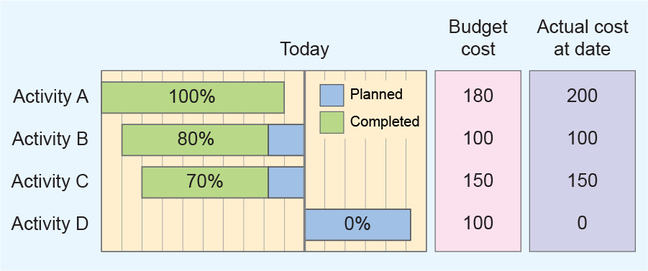4.4

# Calculate earned value measuresIt is now time to apply earned value analysis to a project.

Three dimensions need to be calculated in earned value analysis:

• the Budgeted Cost of the Work Scheduled (BCWS) (or Planned Value)
• the Budgeted Cost of the Work Performed (BCWP) (or Earned Value)
• the Actual Cost of the Work Performed (ACWP).

The planned value, or BCWS, quantifies how much work you planned to have accomplished at a given point in time. It is the sum of the budget costs of all the activities planned to be done till that date.

The earned value, or BCWP, is the budget value of what you have accomplished at a given point in time. In other words, the budget cost of what you have actually done. It is given by the budget cost of the activity times the percentage of accomplishment at that date.

The ACWP represents how much you have actually spent at a given point in time: